Courses

# Test: Adsorption - 2

## 30 Questions MCQ Test Physical Chemistry | Test: Adsorption - 2

Description
This mock test of Test: Adsorption - 2 for Chemistry helps you for every Chemistry entrance exam. This contains 30 Multiple Choice Questions for Chemistry Test: Adsorption - 2 (mcq) to study with solutions a complete question bank. The solved questions answers in this Test: Adsorption - 2 quiz give you a good mix of easy questions and tough questions. Chemistry students definitely take this Test: Adsorption - 2 exercise for a better result in the exam. You can find other Test: Adsorption - 2 extra questions, long questions & short questions for Chemistry on EduRev as well by searching above.
QUESTION: 1

Solution:
QUESTION: 2

Solution:
QUESTION: 3

### The mole of gases NH3, CO2 and H2 adsorbed by 1 g charcoal at 300 K and 1 atm pressure shows the order:

Solution:
QUESTION: 4

Variation of x/m vs. P are plotted for a gas at different temperature as shown below. The correct order of temperature is: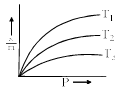Solution:
QUESTION: 5

Methylene blue, from its aqueous solution, is adsorbed on activated charcoal at 25°C. For this process, the correct statement is:

Solution:
QUESTION: 6

Adsorption of gas on solid surface depends upon:

Solution:
QUESTION: 7

Which of the following statements is correct for the spontaneous adsorption of a gas:

Solution:
QUESTION: 8

3 g of activated charcoal was added to 50 mL of acetic acid solution (0.06 N) in a flask. After an hour it was filtered and the strength of the filtrate was found to be 0.042 N. The amount of acetic acid adsorbed (per gram of charcoal) is:

Solution:
QUESTION: 9

For a linear plot of log (x/m) versus log P in a Freundlich adsorption isotherm, which of the following statements is correct (k and n are constants):

Solution:
QUESTION: 10

Charcoal (1 g) of surface area 100 m2 per gram, adsorbs 60 mg of acetic from an aqueous solution at 25°C and 1 atmosphere pressure. The number of moles of acetic acid adsorbed per cm2 of charcoal surface is:

Solution:
*Multiple options can be correct
QUESTION: 11

Which statements are correct:

Solution:
*Multiple options can be correct
QUESTION: 12

The correct statement(s) pertaining to the adsorption of a gas on a solid surface is (are):

Solution:
1. When the gas adsorb surface energy decreases which appears in the form of heat i.e. exothermic.

2. At high temperature gas molecules get activation energy required to form chemical bond and hence chemisorption is favored.

3. As per le chatlier principle physiosorption being exothermic should decrease with temperature.

4. Obviously reactions that require high activation energy are slow.

Hence A, B and D are correct.

*Multiple options can be correct
QUESTION: 13

The given graphs/data I, II, III and IV represent general trends observed for different physisorption and chemisorption process under mild conditions of temperature and pressure. Which of the following choice(s) about I, II III and IV is (are) correct: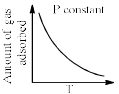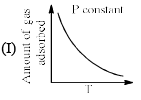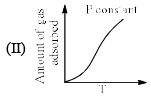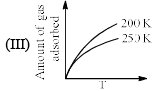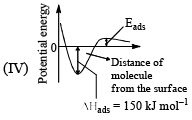Solution:
QUESTION: 14

Which of the followings is not correctly matched:

Solution:
*Multiple options can be correct
QUESTION: 15

Which of the followings are favourable conditions for physical adsorption:

Solution:
*Multiple options can be correct
QUESTION: 16

Which of the following interface can exist:

Solution:
*Multiple options can be correct
QUESTION: 17

Select the correct statements:

Solution:
*Multiple options can be correct
QUESTION: 18

True statement(s) about Langmuir isotherm is (are):

Solution:
*Multiple options can be correct
QUESTION: 19

When O2 is adsorbed on a metallic surface, electron transfer occurs from the metal to O2. The true statement(s) regarding this adsorption is(are):

Solution:
*Multiple options can be correct
QUESTION: 20

According to Freundlich adsorption isotherm which of the following is correct:

Solution: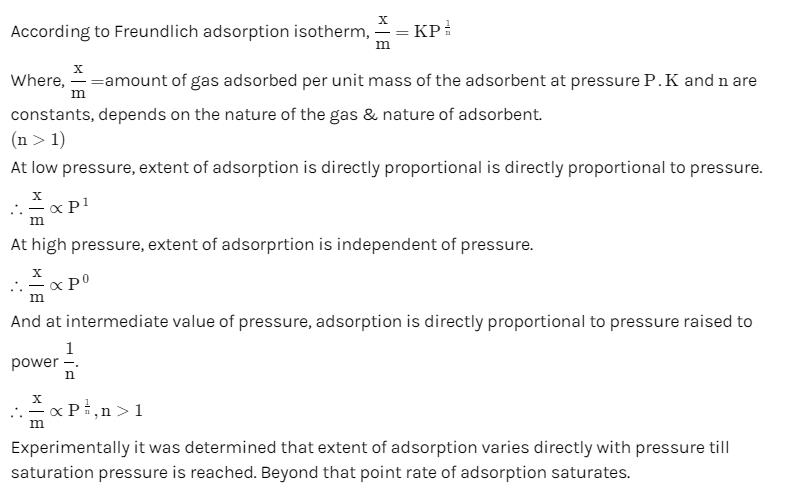*Answer can only contain numeric values
QUESTION: 21

The adsorption of a gas follows the Langmuir isotherm with K = 1.25 kPa–1 at 25°C. The pressure (in Pa) at which surface coverage is 0.2 is..............

Solution:
*Answer can only contain numeric values
QUESTION: 22

Calculate the surface area of a catalyst that adsorbs 103 cm3 of nitrogen reduced to STP per gram in order to form the monolayer. The effective area occupied by N2 molecule on the surface is 1.62 × 10–15 cm2.

Solution:
*Answer can only contain numeric values
QUESTION: 23

1 g of activated charcoal has a surface area 103 m3. If complete monolayer coverage is assumed and effective surface area of NH3 molecule is 0.129 cm2, how much NH3 in cm3 at STP could be adsorbed on 25 g of charcoal.

Solution: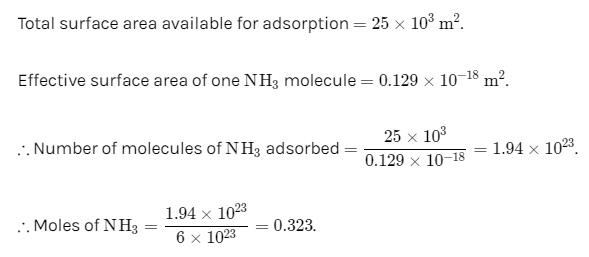*Answer can only contain numeric values
QUESTION: 24

1 g of activated charcoal has a surface area 103 m3. If complete monolayer coverage is assumed, how much NH3 in cm3 at STP would be adsorbed on the surface of 25 g of the charcoal. Given diameter of NH3 molecule = 0.3 nm

Solution:
*Answer can only contain numeric values
QUESTION: 25

20% surface sites have absorbed N2. On heating N2 gas is evolved from sites and were collected at 0.001 atm and 298 K in a container of volume 2.46 cm3. Density of surface sites is 6.023 × 1014 cm–2 and surface area is 1000 cm2. Find out the number of surface sites occupied per molecule of N2.

Solution:
QUESTION: 26

1 g of charcoal adsorbs 100 mL of 0.5 MCH3COOH to form a monolayer and thereby the molarity of CH3COOH reduces to 0.49. Calculate the surface area of charcoal adsorbed by each molecule of acetic acid. Surface area of charcoal = 3.01 × 102 m2/g.

Solution:
QUESTION: 27

H2 gas was adsorbed on 1 g powdered copper surface forming monolayer of molecules. On desorption total H2 collected measured 1.36 cm3 at STP. Assuming volume of 1 molecule of H2 4.742 × 10–23 cm3, calculate specific area of copper powder

Solution:
*Answer can only contain numeric values
QUESTION: 28

A graph plotted between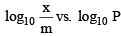(P is pressure of gas in atm) shows a straight line with slope equal to 1 and intercept equal to 0.4771. The extent of adsorption (x/m) of gas at the pressure 2 atm is:

Solution:
*Answer can only contain numeric values
QUESTION: 29

Adsorption of ethanoic acid on wood charcoal follows Freundlich isotherm. Calculate the mass of ethanoic acid adsorbed by 500 g of wood charcoal at 300 K form 3 litre of 0.65 M ethanol solution. The value of constant k = 0.16 and n = 2.35. Also report the molarity of left ethanol in solution.

Solution:
*Answer can only contain numeric values
QUESTION: 30

After placing 9 g of charcoal in a one litre vessel containing gas at 300 K, the pressure of the gas decreases by 40% of the original pressure equal to 700 milli bar. Calculate the volume of gas at 1 bar and 273.15 K adsorbed per g of charcoal. Density of charcoal sample is 1.5 g cm–3.

Solution: